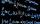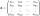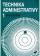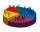Derivation

Exists a function whose derivation is the same function?

Result

Solution:Leave us a comment of example and its solution (i.e. if it is still somewhat unclear...):Be the first to comment!Next similar examples:

1. Minimum of sumFind a positive number that the sum of the number and its inverted value was minimal.Which of the points belong function f:y= 2x2- 3x + 1 : A(-2, 15) B (3,10) C (1,4)
3. Sequence - 5 membersWrite first five members of the sequence ?
4. The inverseThe inverse matrix for matrix A has a determinant value of 0.333. What value has a determinant of the matrix A?
5. AsymptoteWhat is the vertical asymptote of ?
6. The determinantThe determinant of the unit matrix equals 7. Check how many rows the A matrix contains.
7. Inverse matrixFind how many times is the larger determinant is the matrix A, which equals 9 as the determinant of its inverse matrix.
8. Owner of the companyThe owner of the company with extensive administrative activity wants to sell the obsolete 3 machines at the price of CZK 1000 per 1 machine with a six-month warranty period. If the machine breaks down during this period, the owner will return the employe
9. Theorem proveWe want to prove the sentence: If the natural number n is divisible by six, then n is divisible by three. From what assumption we started?
10. BallsWe have n identical balls (numbered 1-n) is selected without replacement. Determine 1) The probability that at least one tensile strength number coincides with the number of balls? 2) Determine the mean and variance of the number of balls, which coincides.
11. Complex number coordinatesWhich coordinates show the location of -2+3i
12. Median and modusRadka made 50 throws with a dice. The table saw fit individual dice's wall frequency: Wall Number: 1 2 3 4 5 6 frequency: 8 7 5 11 6 13 Calculate the modus and median of the wall numbers that Radka fell.Find the roots of the quadratic equation: 3x2-4x + (-4) = 0.Determine the quadratic equation absolute coefficient q, that the equation has a real double root and the root x calculate: ?Equation ? has one root x1 = 8. Determine the coefficient b and the second root x2.Solve two equations with two unknowns: 400x+120y=147.2 350x+200y=144Calculate the Euclidean distance between shops A, B and C, where: A 45 0.05 B 60 0.05 C 52 0.09 Wherein the first figure is the weight in grams of bread and second figure is price in USD.